Gravitation of the planets and its effect on the stock market

Case study

written by Sergey Tarassov

As definition states: "gravitation force is the tendency of objects with mass to accelerate toward each other" (see http://en.wikipedia.org/wiki/Gravity/). This force makes the Moon moving around the Earth, and the Earth and other planets moving around the Sun. This force keeps Galaxies together preventing their dissipation on single planets and planets' dissipation on single atoms.

For physicists, the gravitation is the most enigmatic force in respect to the other knowing forces.

We can do a research on the impact of this force on the stock market. In this small article I will show how to do it for Dow Jones Industrial Index 1885-2006 with Timing Solution software.

In Timing Solution, the gravitational force is presented by these three persons: a gravitational force itself, a tidal force and a gravitational potential. We will explain the difference between them using the classical legend of how Sir Isaac Newton had got this idea observing a falling apple.

Gravitational force: it is the degree of how the Earth accelerates the apple.

Tidal force: different parts of our apple are affected by the Earth differently. The side of the apple that is closer to the Earth is affected stronger than the other side of it. For the apple, this difference is miserable, but for the astronomical scale this force becomes very important.

Gravitation potential: we can take it as the energy that apple is getting when it falls.

In Timing Solution, define the gravitation terms here: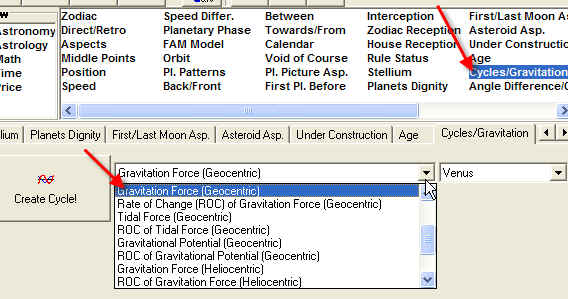As an example, let us consider the planet of Venus. First of all, we want to know the gravitation force of Venus that affects the habitants of the Earth.

Set these options: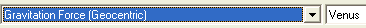Here is the diagram for this force in 2006-2009: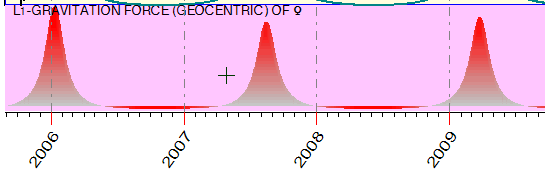This is the oscillation of Venus gravitation around its average value.

Sometimes it may be more interesting to deal with the change of this force in time, not with the gravitation force itself. The force may be small, while its change is pretty significant.

In this case, we should research Rate Of Change (ROC) of Gravitation force, it is here: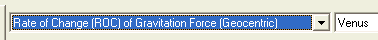For the same time period, ROC of the gravitation force looks like this: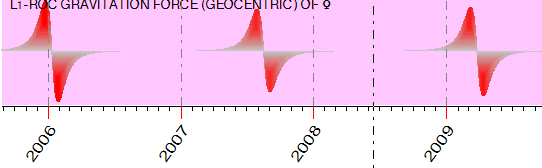You may choose to see the tidal force and the gravitational potential; these options are here as well: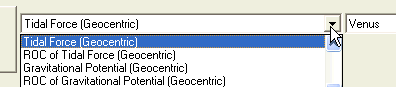Actually the gravitation, the tidal force and the potential look pretty much the same: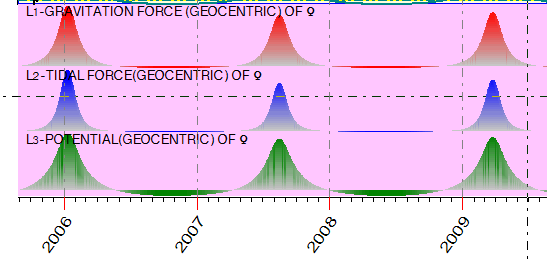The most narrow peak is for Venus tidal force.

This has been done for Venus gravitation toward the Earth. We can do the same for the gravitation between Venus and the Sun. Set these parameters: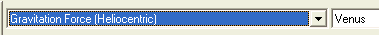"Heliocentric" means that we consider the effect of the planets towards the Sun, not the Earth. The gravitation force of Venus on the Sun looks like this: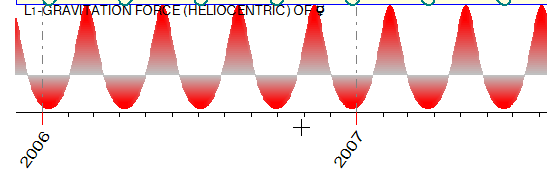Change of this gravitation force looks very interesting:Very similar pictures are received for the tidal force and the gravitational potential.

Now, let us research how these forces affect the Dow Jones Industrial Index (DJI). I have downloaded the available history, from 1885 to 2006.

Click this button:to set Learning Border Cursor to the last point. Thus we will use the whole price history to do the analysis.

We start with the gravitation force of Mercury: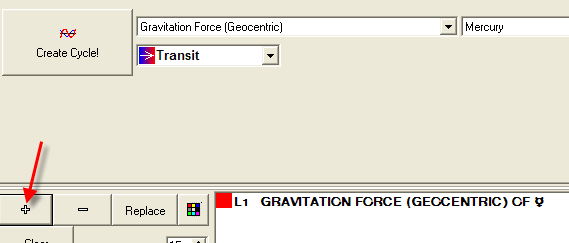Calculate this event ("Calculate" button) and click "R" button: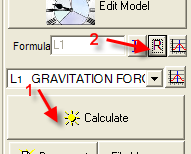You will get this information window: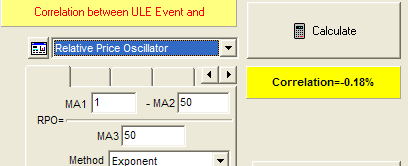The correlation -0.18%  is pretty small, so we can conclude that Mercury gravitation does not affect the DJI movement.

Checking all  possibilities one by one, I have found the following important phenomena:

a) Gravitation force of Venus (geocentric). Correlation is about 5%: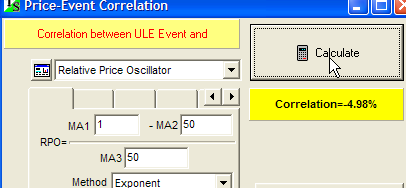The negative correlation means that the DJI tends to reach its LOW point when Venus gravitation is high: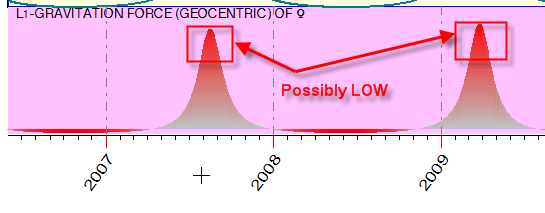b) Changes of Mars gravitation force (geocentric). Correlation  is  2.3%, not big. Practically the same results are received for the changes of Mars tidal force.

Here is its effect:c) Very strong influence is provided by Mars gravitation force in Heliocentric coordinates. I have got here the biggest negative correlation -7.9%. The negative correlation means the inversion - when tops of the diagram correspond to the bottoms of DJI, and vice versa.

The wave provided  by this event looks: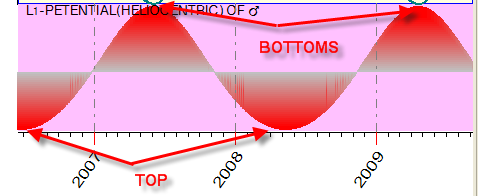Actually, the impact on DJI of the planetary gravitation does not look significant (except Mars's). However, I have got some not bad Neural Net projection line based on gravity effects: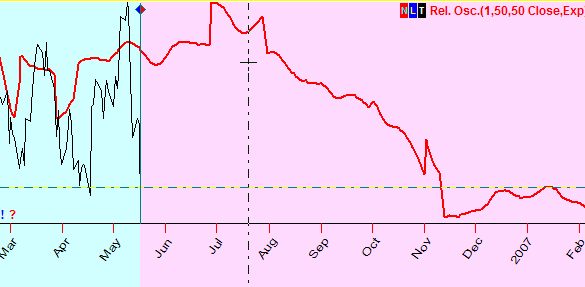In Neural Net module, you can define the gravitational effects here:And the last note: it looks like the gravitational forces are more effective for non directional indicators such as volatility.

This issue needs additional research on different financial instruments. For example, the volatility of gold price has a big correlation to the changes of Mercury's gravity force (geocentric):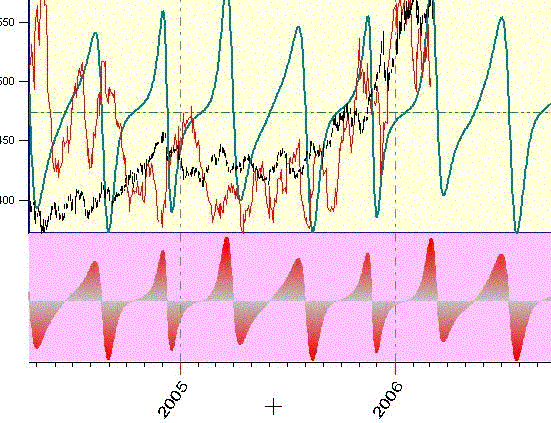Or, if we do a negative sum of the effects of gravity forces for the Sun, Mercury, Venus, Mars and Jupiter: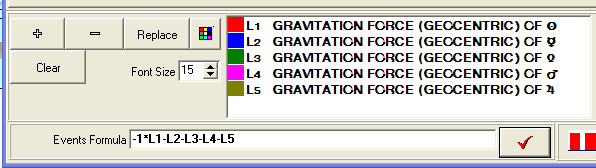,

we will get a very good approximation for ADX index (this fact is proved with probability 98%):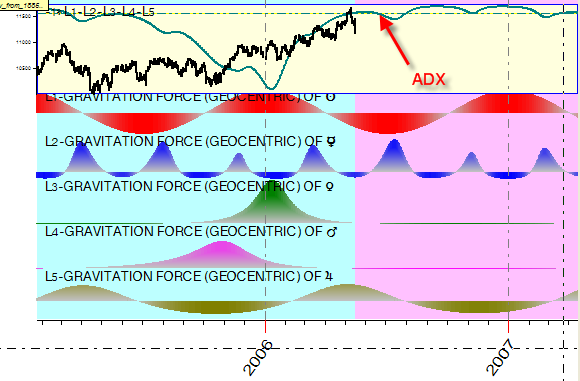I hope I have shown the possible frame of this research.